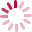# Digital Signal Processing

• Cours
• 4 crédits

Hmaied SHAIEK

## Public, conditions d’accès et prérequis

• Have, at least, bac + 2 level in electronics (BTS, DUT, DPCT).

## Objectifs pédagogiques

• Analog to digital signal conversion concept,
• Sampling and analog to digital conversion
• Discrete signal Fourier and FFT analysis,
• FIR and IIR filtering,
• Introduction to random signal processing,

## Compétences visées

• Provide the basics of digital signal processing, making the connection between theoretical analysis and applications.
• Be able to design and implement a processing device or software.
• Design and build a digital signal processing device.

## Contenu

Learning OutcomesBy the end of this course, the students will be able to:
• characterize the main elements of a digital signal processing scheme, including ADC, DAC, anti-aliasing filter and reconstruction filter,
• compute the discrete Fourier transform, the z-transform of discrete signal,
• implement digital filters of the FIR or IIR type, and study the stability and the phase behavior of digital filters,
• compute the auto-correlation, cross correlation and PSD of discrete random signals,
• understand the need for designing an adaptive digital filter with coefficients computed based on LMS or RLS algorithm.
• use Matlab for simulations of digital signal processing.
Items
• Introduction
• Discrete Fourier transform, FFT and z-transform,
• FIR filtering
• Design of FIR filters and applications
• IIR filtering
• Design of IIR filters and applications
• Introduction to discrete random signal processing,
• Project: students will be asked to put in practice assimilated notions to carry out a project describing a practical application. This project will be developed using MATLAB and dedicated digital signal processing platforms.

## Modalité d'évaluation

• Project mark plus final exam.
Project (40%) and Exam (60%).

## Bibliographie

• A.V Oppenheim and R.W. Schafer : Discrete Time Signal Processing, Pearson New International Edition, 3rd edition, 2014.
• J. G. Proakis and D. G. Manolakis : Digital Signal Processing: Principles, Algorithms, and Applications, Prentice Hall International, 3rd edition 1996.

## Cette UE apparaît dans les diplômes et certificats suivants

Chargement du résultat...Intitulé de la formation
Type
Modalité(s)
Lieu(x)
Lieu(x) Initial
Lieu(x) Paris
Intitulé de la formation Type Modalité(s) Lieu(x)

## Contact

EPN03 - Easy
292 rue Saint-Martin 11-B-2
75141 Paris Cedex 03
Tel :01 40 27 24 81